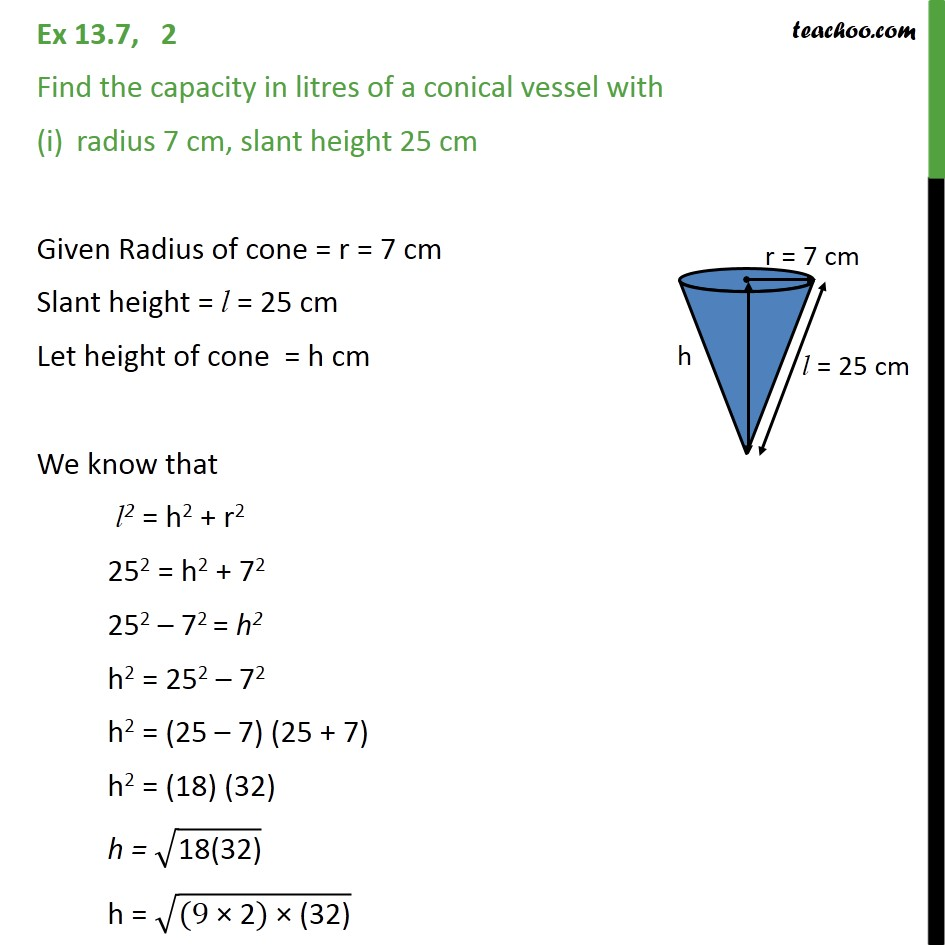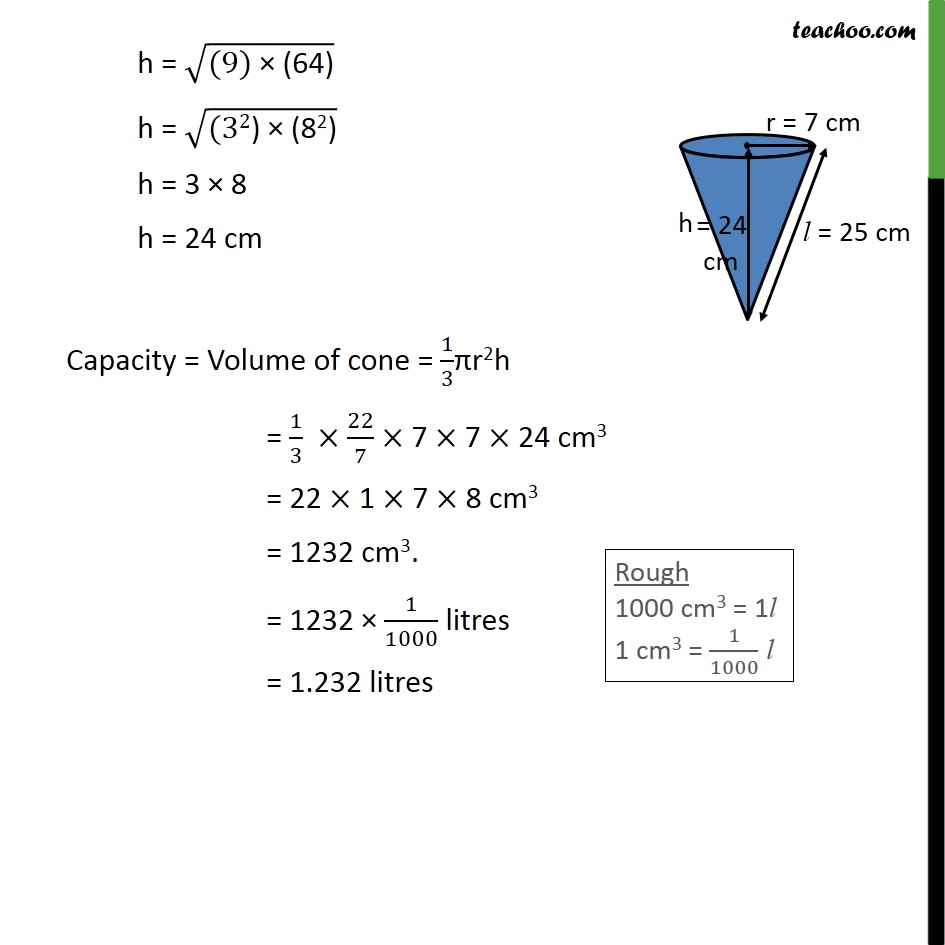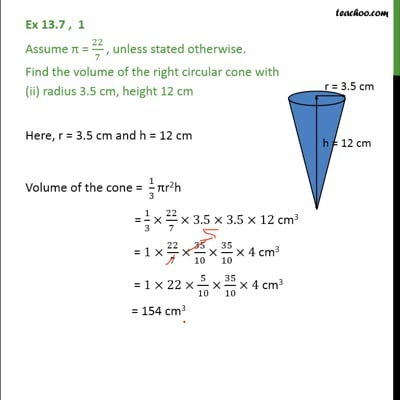Volume Of Cone

Chapter 13 Class 9 Surface Areas and Volumes
Concept wiseThis video is only available for Teachoo black users

Introducing your new favourite teacher - Teachoo Black, at only ₹83 per month

### Transcript

Ex 13.7, 2 Find the capacity in litres of a conical vessel with radius 7 cm, slant height 25 cm Given Radius of cone = r = 7 cm Slant height = l = 25 cm Let height of cone = h cm We know that l2 = h2 + r2 252 = h2 + 72 252 – 72 = h2 h2 = 252 – 72 h2 = (25 – 7) (25 + 7) h2 = (18) (32) h = √("18(32)" ) h = √("(9 × 2) × (32)" ) h = √("(9) × (64)" ) h = √("(32) × (82)" ) h = 3 × 8 h = 24 cm Capacity = Volume of cone = 1/3πr2h = 1/3 × 22/7 × 7 × 7 × 24 cm3 = 22 × 1 × 7 × 8 cm3 = 1232 cm3. = 1232 × 1/1000 litres = 1.232 litres